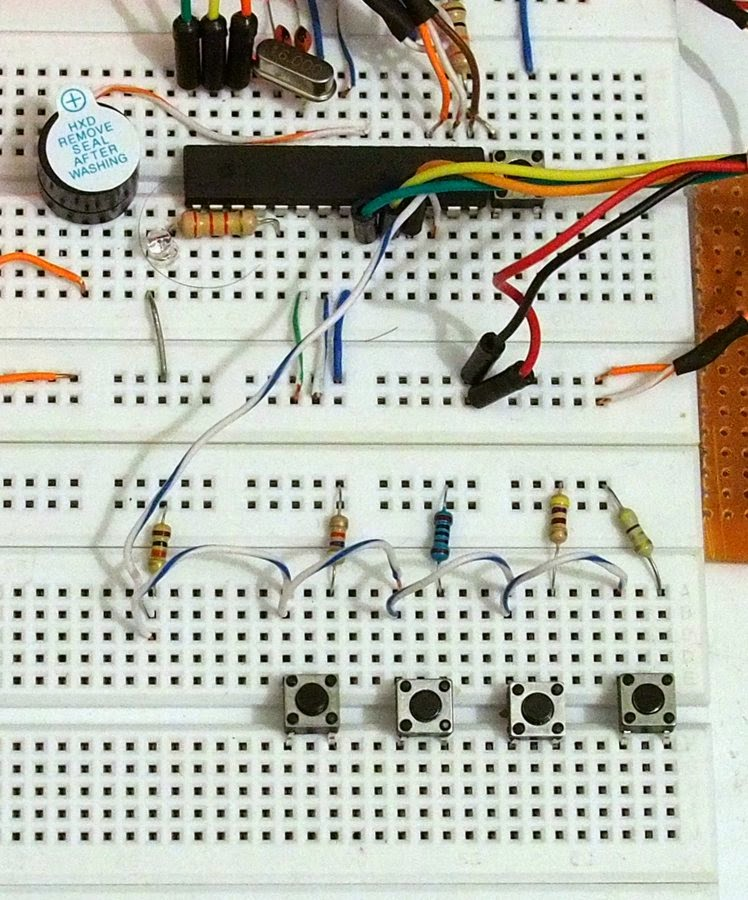### Multi buttons input for single analog pin on Arduino

For triple NTC-thermistor probes (part1) access here.

Hi again, today I continue my little experimentation with Arduino. The ultimate aim is to have a homemade incubator. This article will discuss a way to have a user input for some parameters in my incubator, like upper temperature limit (upperTemp), lower temperature limit (lowerTemp), heating and cooling time during the normal operation (heatingTime and coolingTime, respectively).

### HARDWARE SETUP

To enable user input, I selected four push buttons, representing select menu,  down, up, and mute buttons.  Learning from an Arduino page, it is fairly simple to understand the logic of multi buttons input for a single analog pin. I employed four different resistors to give different input values to an Arduino analog pin.

For this,  I prepared electronic parts:

1. 4x four-legged push buttons,
2. 1x 10kΩ, 1x 4.7 kΩ, 1x 1kΩ, 1x 470Ω, and 1x 47Ω resistors,
3. Some jumper cables

###PROGRAMMING

#define keyPin A3
#define btnNone 0
#define btnSelect 1
#define btnDown 2
#define btnUp 3
#define btnMute 4
void setup()
{
//Serial connection
Serial.begin(9600);
//initialize pinouts
pinMode(keyPin, INPUT);
}
void loop()
{
int keypressed=0;
keypressed = keypress();
}
int keypress()
{
int value;
int btnValue;

//debugging
Serial.print("Value: "); Serial.println(value);
}

From this I collected return value for each button, hence

1. btnNone return value > 1000
2. btnSelect return value < 350, R=4k7Ω
3. btnDown return value < 80, R=1k Ω
4. btnUp return value < 30, R=470 Ω
5. btnMute return value < 10, R=47 Ω

Then I appended the keypress function with these lines:
int keypress()
{
...
//VCC----[R 10k]--[Analog Pin]---[selected R]--GND
//btnNone return value >  1000
//btnSelect return value <  350, R=4k7
//btnDown return value <  80, R=1k
//btnUp return value <  30, R=470
//btnMute return value <  10, R=47

if (value> 1000) {
btnValue=0;  //btnNone
} else if (value <  10) {
btnValue=4;  //btnMute
} else if (value <  30) {
btnValue=3;  //btnUp
} else if (value <  80) {
btnValue=2;  //btnDown
} else if (value <  350) {
btnValue=1;  //btnSelect
}
//debugging
//Serial.print("btnValue: "); Serial.println(btnValue);
return btnValue;
}

Since I have four parameters to be adjusted: upperTemp, lowerTemp, heatingTime, and coolingTime, therefore I need to create at least four setup sub-menu and another one for saving all parameters either to internal Arduino memory space (EEPROM) or to an external (micro)SD card.

My  incubator will not operate sub-zero or more than 80, than I need to put some logics on the code. Also, heating and cooling time should be in between 1 – 99.

//running mode
/*
0 = default;
1 = setup upperTemp;
2 = setup lowerTemp;
3 = setup heatingTime;
4 = setup coolingTime;
5 = save all changes (Y/N);
*/
int runMode=0;

{
int keypressed=0;
keypressed = keypress();

switch (keypressed) {
case btnMute: {
tactile();
break;
} case btnSelect: {
if (runMode==0) {
runMode=1;
} else if (runMode==1) {
runMode=2;
} else if (runMode==2) {
runMode=3;
} else if (runMode==3) {
runMode=4;
} else if (runMode==4) {
runMode=5;
} else {
runMode=0;
}
tactile();
break;

} case btnDown: {
if (runMode==1) {
if (upperTemp> (lowerTemp+1)) {
upperTemp -= 1;
}
} else if (runMode==2) {
if (lowerTemp> 1) {
lowerTemp -= 1;
}
} else if (runMode==3) {
if (heatingTime> 1) {
heatingTime -= 1;
}
} else if (runMode==4) {
if (coolingTime> 1) {
coolingTime -= 1;
}
} else {
runMode=0;
}
tactile();
break;
} case btnUp: {
if (runMode==1) {
if (upperTemp< 80) {
upperTemp += 1;
}
} else if (runMode==2) {
if (lowerTemp< (upperTemp-1)) {
lowerTemp += 1;
}
} else if (runMode==3) {
if (heatingTime< 98) {
heatingTime += 1;
}
} else if (runMode==4) {
if (coolingTime< 98) {
coolingTime += 1;
}
} else if (runMode==5) {
//writeSetting, yet to be done;
}
tactile();
break;

} case btnNone: {
break;
}
}
}

I added a simple tactile function to determine best delay for easiness of use by user.

void tactile()
{
msgSelectState(runMode);
delay(1000);
}

I called another function (msgSelectState) in which I stored all human-readable message either to a LCD, LED, 7 segment, or in my case to serial connection.

void msgSelectState(int mode)
{
if (mode==1) {
//do something elese here

//debugging
Serial.print("Setup, upperTemp: "); Serial.println(upperTemp);
} else if (mode==2) {
//do something elese here
//debugging
Serial.print("Setup, lowerTemp: "); Serial.println(lowerTemp);
} else if (mode==3) {
//do something elese here
//debugging
Serial.print("Setup, heatingTime *5s: "); Serial.println(heatingTime);
} else if (mode==4) {
//do something elese here
//debugging
Serial.print("Setup, coolingTime *5s: "); Serial.println(coolingTime);
} else if (mode==5) {
//do something elese here
//debugging
Serial.println("Save Setting? UP(Y) DWN(N)");
} else {
//do something elese here
//debugging
Serial.println("running");
}
}

Now it is time to combine everything together. Yo may download the whole code here
You may visit another implementation of multiple buttons on single pin from Tronixstuff.

### TIME FOR FUN

Lets connect the FTDI cable to a computer USB port.

Enjoy!